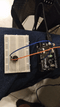To this point we have controlled light, motion, and electrons. Let’s tackle sound next. But sound is an analog phenomena, how will our digital Arduino cope? We will once again rely on its incredible speed which will let it mimic analog behavior. To do this, we will attach a piezo element to one of the Arduino’s digital pins. Piezo buzzers are simple devices that can generate basic beeps and tones.  They work by using a piezo crystal, a special material that changes shape when voltage is applied to it.  If the crystal pushes against a diaphragm, like a tiny speaker cone, it can generate a pressure wave which the human ear picks up as sound.  Simple change the frequency of the voltage sent to the piezo and it will start generating sounds by changing shape very quickly! Let’s get to experimenting with it and get your Arduino playing “Twinkle Twinkle Little Star”.

### Hardware Used:

• 1 – Piezo Buzzer

• 1 – Arduino UNO
•

•

You can buy all this Hardware at Createlabz.

Arduino IDE

### Set up the Hardware:### Code:

```/* Melody
* (cleft) 2005 D. Cuartielles for K3
*
* This example uses a piezo speaker to play melodies.  It sends
* a square wave of the appropriate frequency to the piezo, generating
* the corresponding tone.
*
* The calculation of the tones is made following the mathematical
* operation:
*
*       timeHigh = period / 2 = 1 / (2 * toneFrequency)
*
* where the different tones are described as in the table:
*
* note   frequency   period  timeHigh
* c          261 Hz          3830  1915
* d          294 Hz          3400  1700
* e          329 Hz          3038  1519
* f          349 Hz          2864  1432
* g          392 Hz          2550  1275
* a          440 Hz          2272  1136
* b          493 Hz          2028  1014
* C          523 Hz          1912  956
*
* http://www.arduino.cc/en/Tutorial/Melody
*/

int speakerPin = 9;

int length = 15; // the number of notes
char notes[] = "ccggaagffeeddc "; // a space represents a rest
int beats[] = { 1, 1, 1, 1, 1, 1, 2, 1, 1, 1, 1, 1, 1, 2, 4 };
int tempo = 300;

void playTone(int tone, int duration) {
for (long i = 0; i < duration * 1000L; i += tone * 2) {
digitalWrite(speakerPin, HIGH);
delayMicroseconds(tone);
digitalWrite(speakerPin, LOW);
delayMicroseconds(tone);
}
}

void playNote(char note, int duration) {
char names[] = { 'c', 'd', 'e', 'f', 'g', 'a', 'b', 'C' };
int tones[] = { 1915, 1700, 1519, 1432, 1275, 1136, 1014, 956 };

// play the tone corresponding to the note name
for (int i = 0; i < 8; i++) {
if (names[i] == note) {
playTone(tones[i], duration);
}
}
}

void setup() {
pinMode(speakerPin, OUTPUT);
}

void loop() {
for (int i = 0; i < length; i++) {
if (notes[i] == ' ') {
delay(beats[i] * tempo); // rest
} else {
playNote(notes[i], beats[i] * tempo);
}

// pause between notes
delay(tempo / 2);
}
}```

### Code Breakdown:

`int speakerPin = 9;`

We assigned the pin of the speaker into the 9.

`int length = 15;`

We assign how many number of notes we will use.

```char notes[] = "ccggaagffeeddc "; // a space represents a rest
int beats[] = { 1, 1, 1, 1, 1, 1, 2, 1, 1, 1, 1, 1, 1, 2, 4 };```

So this are the notes that we will use. There is a table about the notes for its frequency, period and timehigh.

```void playTone(int tone, int duration) {
for (long i = 0; i < duration * 1000L; i += tone * 2) {
digitalWrite(speakerPin, HIGH);
delayMicroseconds(tone);
digitalWrite(speakerPin, LOW);
delayMicroseconds(tone);
}```

We made a function playTone(), inside this function there is digitalWrite() code as this will initate the music if this function is called. HIGH means on, LOW means off.

`pinMode(speakerPin, OUTPUT);`

assigning the Pin 9 which is the signal of the Buzzer as an output.

## Try MOREEE!!!

### Twinkle Twinkle Little Star

```int length = 15;
char notes[] = {"ccggaaggffeeddc "};
int beats[] = { 1, 1, 1, 1, 1, 1, 2, 1, 1, 1, 1, 1, 1, 2, 4 };```

### Happy Birthday (first line)

```int length = 13;
char notes[] = {"ccdcfeccdcgf "};
int beats[] = {1,1,1,1,1,2,1,1,1,1,1,2,4};```

### Output:

The post Arduino Starter’s Guide (6/7): Piezo Buzzer appeared first on CreateLabz.